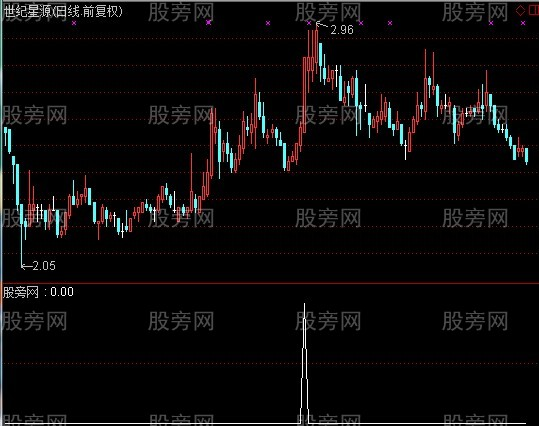# 通达信倍量涨停指标公式

a1:=c/ref(c,1)>1.088 and count(c<ref(c,1) and v>ref(ma(v,135),1)*1.2,10)=0;
a2:=v>ma(v,135)*1.2 and v>ref(v,1) and ref(v,1)<ma(v,135)*2;
a3:=count(v>ref(ma(v,135),1)*1.05,6)<4;
a4:=c<ref(c,1) and v>ref(ma(v,135),1)*1.05;
a5:=count(a4,8)<2 and not(ref(c,1)>ref(c,2) and ref(v,1)>ma(v,120)*1.05 and ref(c,2)>ref(c,3) and ref(v,2)>ma(v,135)*1.05);
a1 and a2 and a3 and a5;